# Aoristic analysis for hour of day and day of week in Excel

I’ve previously written code to conduct Aoristic analysis in SPSS. Since this reaches about an N of three crime analysts (if that even), I created an Excel spreadsheet to do the calculations for both the hour of the day and the day of the week in one go.

Note if you simply want within day analysis, Joseph Glover has a nice spreadsheet with VBA functions to accomplish that. But here I provide analysis for both the hour of the day and the day of the week. Here is the spreadsheet and some notes, and I will walk through using the spreadsheet below.

First off, you need your data in Excel to be `BeginDateTime` and `EndDateTime` — you cannot have the dates and times in separate fields. If you do have them in separate fields, if they are formatting correctly you can simply add your date field to your hour field. If you have the times in three separate date, hour, and minute fields, you can do a formula like `=DATE + HOUR/24 + MINUTE/(60*24)` to create the combined datetime field in Excel (excel stores a single date as one integer).

Presumably at this stage you should fix your data if it has errors. Do you have missing begin/end times? Some police databases when there is an exact time treat the end date time as missing — you will want to fix that before using this spreadsheet. I constructed the spreadsheet so it will ignore missing cells, as well as begin datetimes that occur after the end datetime.

So once your begin and end times are correctly set up, you can copy paste your dates into my `Aoristic_HourWeekday.xlsx` excel spreadsheet to do the aoristic calculations. If following along with my data I posted, go ahead and open up the two excel files in the zip file. In the `Arlington_Burgs.xlsx` data select the B2 cell.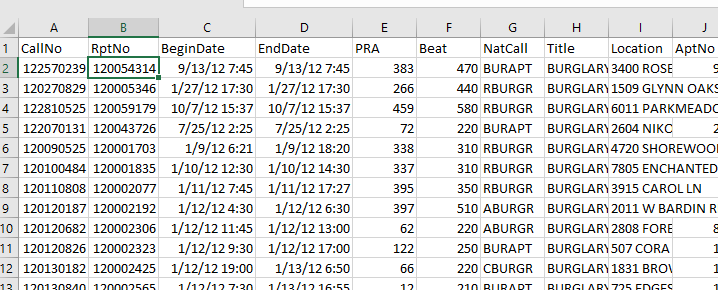Then scroll down to the bottom of the sheet, hold `Shift`, and then select the D3269 cell. That should highlight all of the data you need. Right-click, and the select Copy (or simply `Ctrl` + `C`).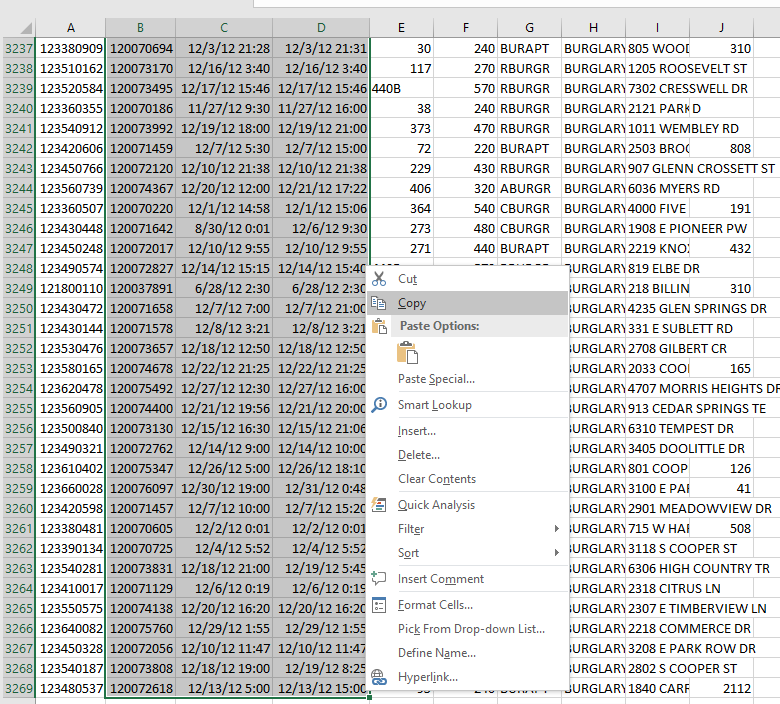Now migrate over to the `Aoristic_HourWeekday.xlsx` spreadsheet, and paste the data into the first three columns of the `OriginalData` sheet.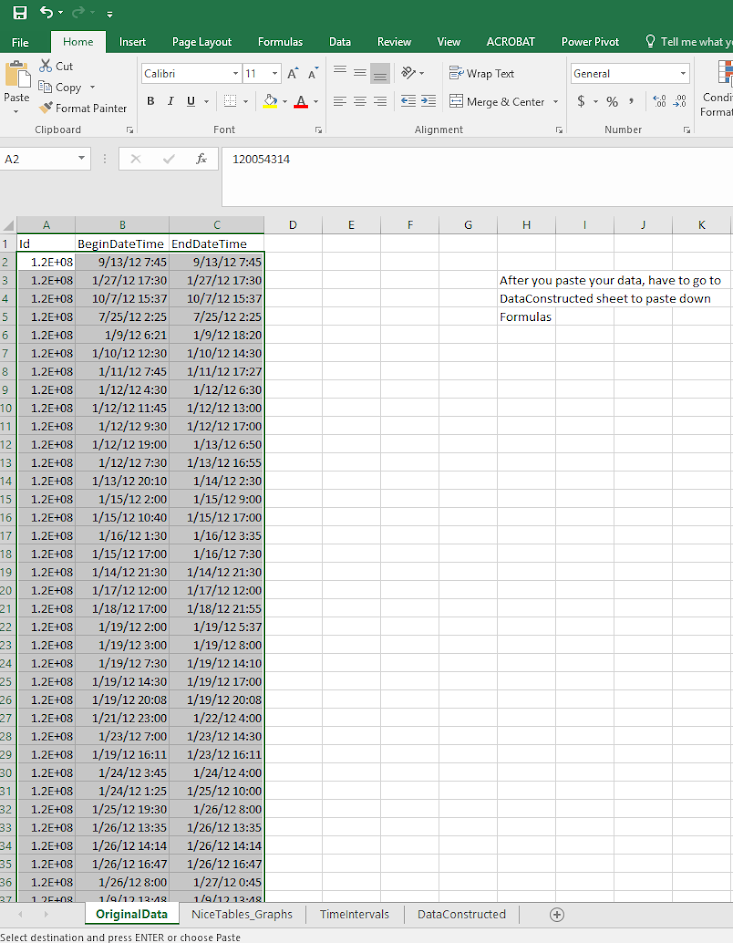Now go to the `DataConstructed` sheet. Basically we need to update the formulas to recognize the new rows of data we just copied in. So go ahead and select the A11 to MI11 row. (Note there are a bunch of columns hidden from view).Now we have a few over 3,000 cases in the Arlington burglary data. Grab the little green square in the lower right hand part of the selected cells, and then drag down the formulas. With your own data, you simply want to do this for as many cases as you have. If you go past your total N it is ok, it just treats the extra rows like missing data. This example with 3,268 cases then takes about a minute to crunch all of the calculations.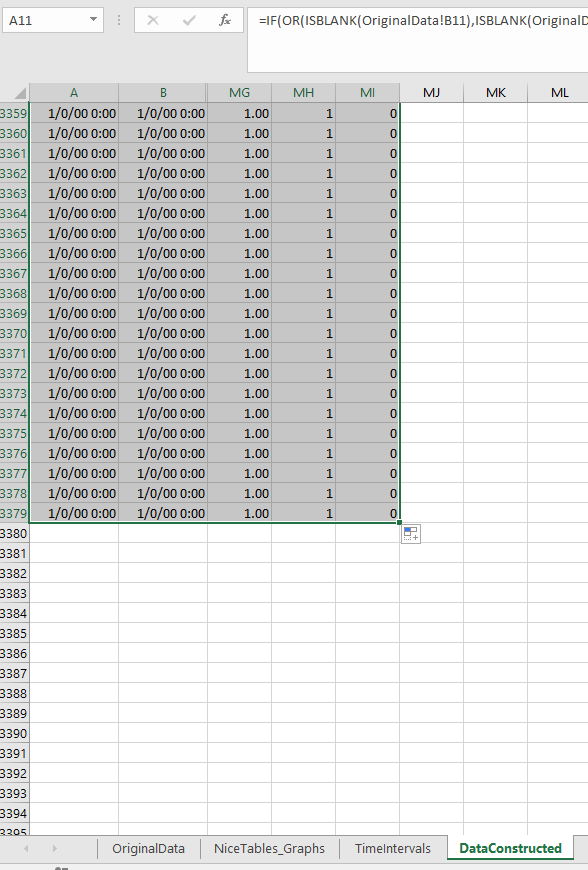If you navigate to the `TimeIntervals` sheet, this is where the intervals are actually referenced, but I also place several summary statistics you might want to check out. The Total N shows that I have 3,268 good rows of data (which is what I expected). I have 110 missing rows (because I went over), and zero rows that have the begin/end times switched. The total proportion should always equal 1 — if it doesn’t I’ve messed up something — so please let me know!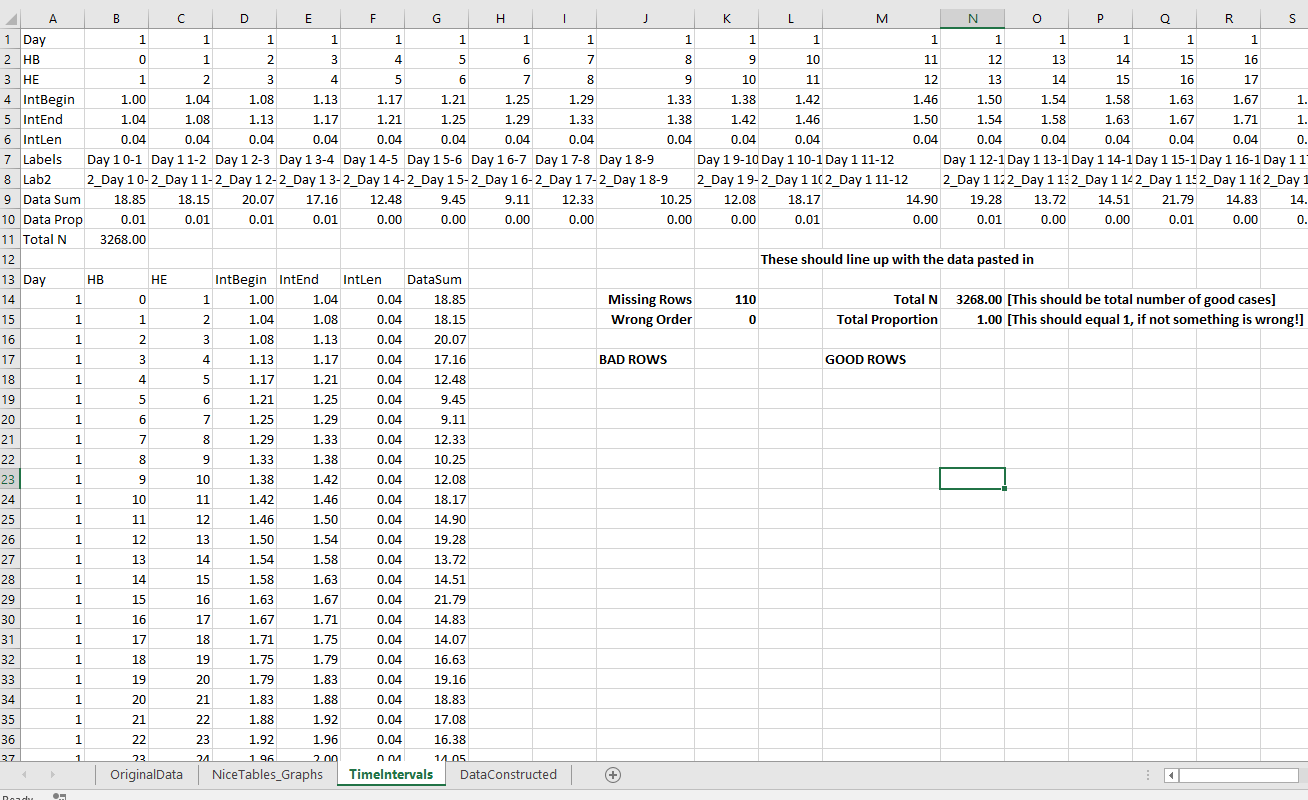Now the good stuff, if you navigate to the `NiceTables_Graphs` sheet it does all the summaries that you might want. Considering it takes awhile to do all the calculations (even for a tinier dataset of 3,000 cases), if you want to edit things I would suggest copying and pasting the data values from this sheet into another one, to avoid redoing needless calculations.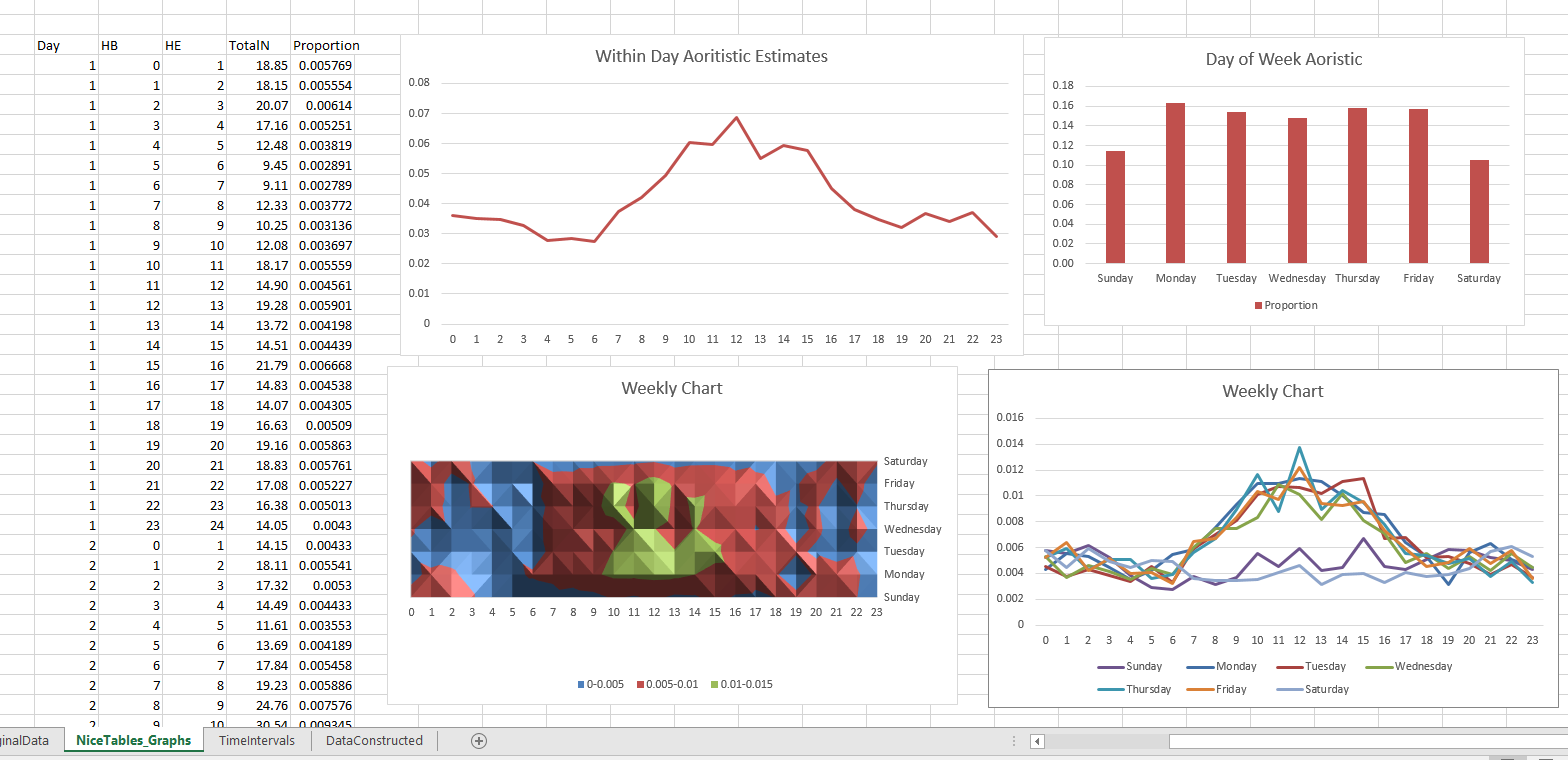Interpreting the graphs you can see that burglaries in this dataset have a higher proportion of events during the daytime, but only on weekdays. Basically what you would expect.

Personally I would always do this analysis in SPSS, as you can make much nicer small multiple graphs than Excel like below. Also my SPSS code can split the data between different subsets. This particular Excel code you would just need to repeat for whatever subset you are interested in. But a better Excel sleuth than me can likely address some of those critiques.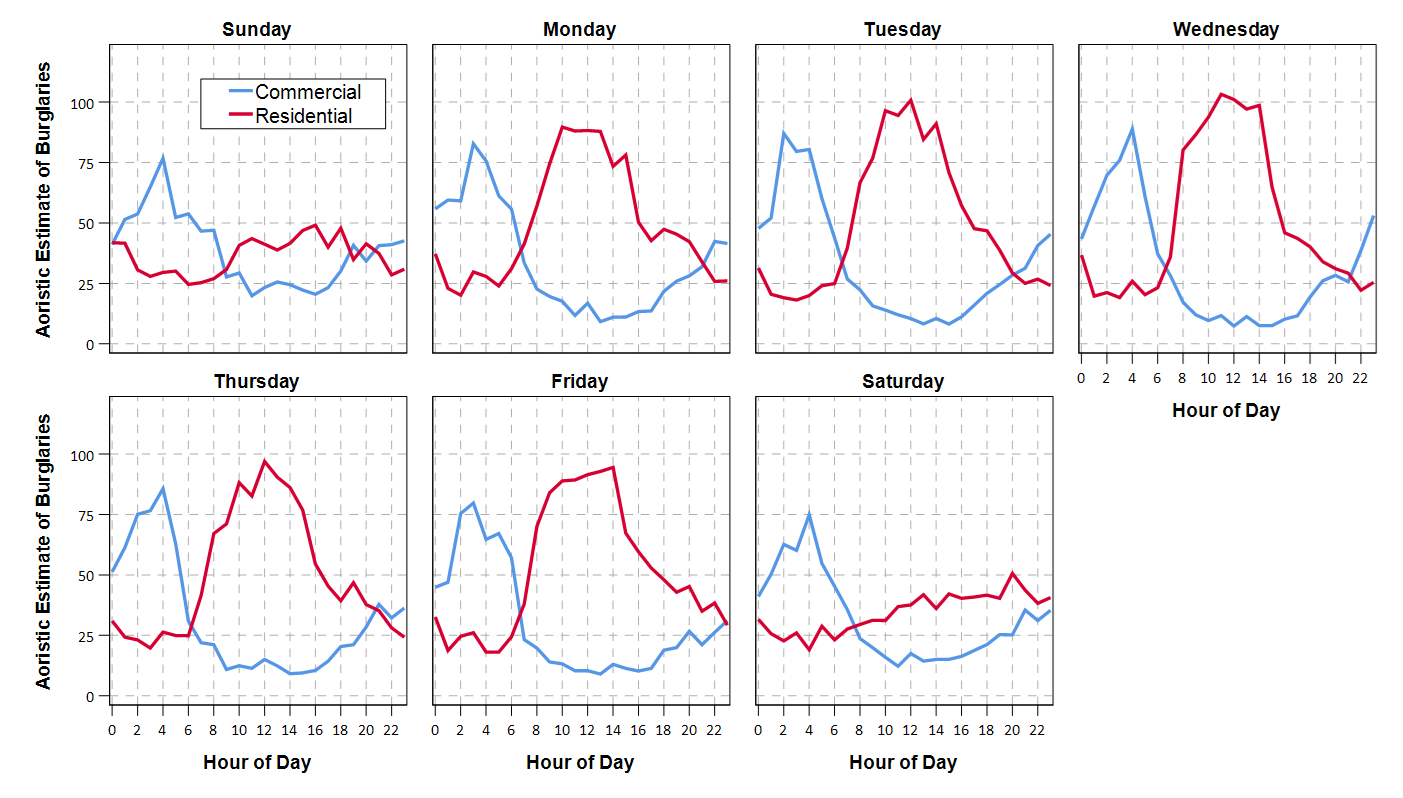One minor additional note on this is that Jerry’s original recommendation rounded the results. My code does proportional allocation. So if you have an interval like 00:50 TO 01:30, it would assign the [0-1] hour as 10/40, and [1-2] as 30/40 (original Jerry’s would be 50% in each hour bin). Also if you have an interval that is longer than the entire week, I simply assign equal ignorance to each bin, I don’t further wrap it around.

1.